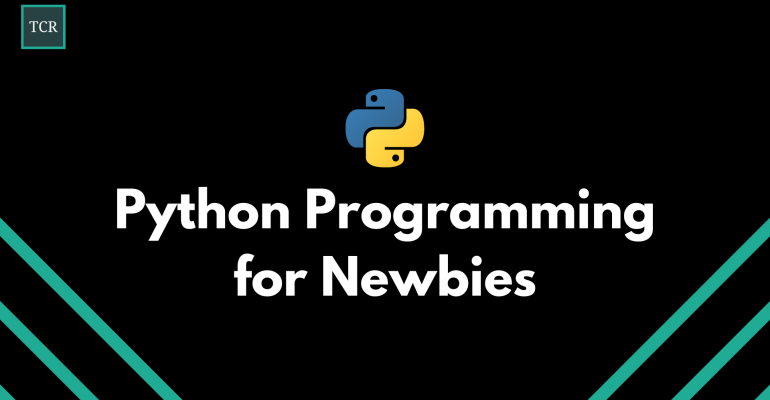Thanks to theidioms.com

## Errors and Exceptions in Python# Errors and Exceptions in PythonIn this lesson, we will learn about when and why various errors and exceptions occur in Python. We will also discuss different ways to handle them.

## Syntax Errors in Python

Syntax errors are errors caused by the use of incorrect Python syntax.

The following example shows an example of a syntax error where the print function was written without any round brackets.

`print "Hello, World!"`
``````OUTPUT:
File "<stdin>", line 1
print "Hello, World!"
^``````

You can see that whenever a syntax error occurs in Python, the parser points at the point where the error has occurred using an upright triangle (^) symbol.

The right way to solve syntax errors is by writing the correct syntax in Python.

## Logical Errors (Exceptions)

Even if a statement or expression is syntactically correct, it may throw out an error during execution. Such errors are termed as logical errors or exceptions.

These errors occur as a result of not correctly writing the logic of the program. The following example shows an exception being raised when a number is divided by zero:

`>>> 10 * (1/0)`
``````OUTPUT:
Traceback (most recent call last):
File "<stdin>", line 1, in <module>
ZeroDivisionError: division by zero``````

As you can see, the parser shows us that there is a exception called `ZeroDivisionError` in line 1.

## How to handle exceptions in Python?

Now that we know what exceptions are, let us learn how to handle them.

Python provides a set of statements that can be used to handle exceptions and they are known as try-except statements. They are similar to try-catch blocks used in other programming languages such as C, C++, and Java.

The syntax for a try-except statement is as follows:

```try:
# Code to be executed by default
except:
# Code to be executed if try block fails to be executed```

The try-except statement consists of two parts: Try and Except. It works as follows:

• The try part of the statement consists of a set of code to be executed.
• If no exception occurs, the code in the try statement block is executed and the except clause is skipped.
• If an exception occurs in any part of the code in the try statement, the rest of the code is skipped and the except statement block is executed.

Here is an example of a try-except statement where we are we are passing the string “dsa” as an input to the program:

```# Simple example of handling exception

try:
x = int(input("Please enter a number: "))
except Exception as e:
print("Oops! There was an exception:", e)    ```
``````OUTPUT:

Oops! There was an exception: invalid literal for int() with base 10: 'dsa'``````

You can note that we’ve generalized the exception occurred with the name ‘e’ in the above program.

We can also specify the type of exception we want to catch using the except statement. Here is an example where the exception is specified as `ValueError` and we are passing the string “dsa” as an input to the program like before:

```# Simple example of handling value error exception

try:
x = int(input("Please enter a number: "))
except ValueError:
print("Oops! That was not a valid number.")```
``````OUTPUT:
Oops! That was not a valid number.``````

Also, if you want to execute something at the end of the try-except statements, then, you can use the try-except-finally statement. The syntax of a try-except-finally statement is as follows:

```try:
# Code to be executed by default
except:
# Code to be executed if try block fails to be executed
finally:
# Code to be executed after the try-except block```

Here is an example showcasing the use of the try-except-finally statement where we are passing the string “dsa” as an input to the program:

```try:
x = int(input("Please enter a number: "))
except Exception as e:
print("Oops! There was an exception:", e)
finally:
print("The finally statement is executed")```
``````OUTPUT:
Oops! There was an exception: invalid literal for int() with base 10: 'dsa'
The finally statement is executed``````

As per the above example, the finally block always executes at the end no matter what exception is raised.

## How to raise exceptions in Python?

Sometimes you might want to raise exceptions by yourself when a program is executing for easier debugging.

You can do so by using the `raise` keyword followed by the type of exception in Python. Here is an example where we are passing -2 as an input to the program below:

```try:
x = int(input("Please enter a positive number: "))
if x < 0:
raise ValueError("Number is negative.")
except Exception as e:
print("Oops! There was an exception:", e)```
``````Please enter a positive number: -2
Oops! There was an exception: Number is negative.``````

In this way, we can raise exceptions by ourselves.

You’ve now understood how to handle errors and exceptions in Python in detail.